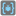• # "The Flat Dictionary" task doesn't accept solution

## Question related to missionThe Flat Dictionary

"""I tested 3 solutions before. And i dont understand, how right solved it."""

```def flatten(dictionary):
stack = [((), dictionary)]
result = {}
while stack:
path, current = stack.pop()
for k,v in current.items():
if isinstance(v, dict) and len(v)>=1:
stack.append((path + (k,),v))
else:
result["/".join((path + (k,)))] = str(v).replace('{}',"")
return result
```